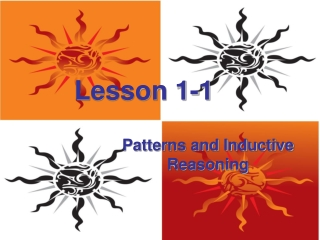DownloadDownload PresentationLesson 1-1

# Lesson 1-1

Télécharger la présentation## Lesson 1-1

- - - - - - - - - - - - - - - - - - - - - - - - - - - E N D - - - - - - - - - - - - - - - - - - - - - - - - - - -
##### Presentation Transcript

1. Lesson 1-1 Patterns and Inductive Reasoning

2. Ohio Content Standards:

3. Ohio Content Standards: • Estimate, compute and solve problems involving real numbers, including ratio, proportion and percent, and explain solutions. -Demonstrate fluency in computations using real numbers. -Estimate, compute and solve problems involving rational numbers, including ratio, proportion and percent, and judge the reasonableness of solutions.

4. Ohio Content Standards: • Estimate, compute and solve problems involving real numbers, including ratio, proportion and percent, and explain solutions. -Demonstrate fluency in computations using real numbers. -Estimate, compute and solve problems involving rational numbers, including ratio, proportion and percent, and judge the reasonableness of solutions. • Generalize and explain patterns and sequences in order to find the next term and the nth term.

5. Vocabulary:

6. Vocabulary: • Inductive Reasoning –

7. Vocabulary: • Inductive Reasoning – When you make a conclusion based on a pattern of examples or past events.

8. Find the next three terms of each sequence.

9. Find the next three terms of each sequence. • 11.2, 9.2, 7.2, …

10. Find the next three terms of each sequence. • 11.2, 9.2, 7.2, … • 6, 12, 24, …

11. Find the next three terms of the sequence:

12. Find the next three terms of the sequence: • 101, 102, 105, 110, 117, …

13. Draw the next figure in the pattern:

14. Draw the next figure in the pattern:

15. Draw the next figure in the pattern:

16. Draw the next figure in the pattern:

17. Vocabulary:

18. Vocabulary: • Conjecture –

19. Vocabulary: • Conjecture – A conclusion that you reach based on inductive reasoning.

20. Vocabulary: • Conjecture – A conclusion that you reach based on inductive reasoning. • Counterexample –

21. Vocabulary: • Conjecture – A conclusion that you reach based on inductive reasoning. • Counterexample – an example that does not follow the conjecture, proving the conjecture false.

22. Jordan studied the data below and made the following conjecture: • Multiplying a number by -1 produces a product that is less than -1.

23. Jordan studied the data below and made the following conjecture: • Multiplying a number by -1 produces a product that is less than -1. 5(-1) = -5 and -5 < -1

24. Jordan studied the data below and made the following conjecture: • Multiplying a number by -1 produces a product that is less than -1. 5(-1) = -5 and -5 < -1 15(-1) = -15 and -15 < -1

25. Jordan studied the data below and made the following conjecture: • Multiplying a number by -1 produces a product that is less than -1. 5(-1) = -5 and -5 < -1 15(-1) = -15 and -15 < -1 100(-1) = -100 and -100 < -1

26. Jordan studied the data below and made the following conjecture: • Multiplying a number by -1 produces a product that is less than -1. 5(-1) = -5 and -5 < -1 15(-1) = -15 and -15 < -1 100(-1) = -100 and -100 < -1 300(-1) = -300 and -300 < -1

27. Jordan studied the data below and made the following conjecture: • Multiplying a number by -1 produces a product that is less than -1. 5(-1) = -5 and -5 < -1 15(-1) = -15 and -15 < -1 100(-1) = -100 and -100 < -1 300(-1) = -300 and -300 < -1 Find a counter example for his conjecture.

28. Assignment: Pgs. 7 – 9 2 – 34 evens